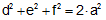Problem 257. Equilateral Triangle, Circumcircle, Point, Vertices, Side, Distances, Squares. Level: High School, College, SAT Prep. The figure shows an equilateral triangle ABC inscribed in a circle. If a point D is on the circumcircle, DA = d, DB = e, DC = f, and AB = a, prove that. View or post a solutionRecent Additions